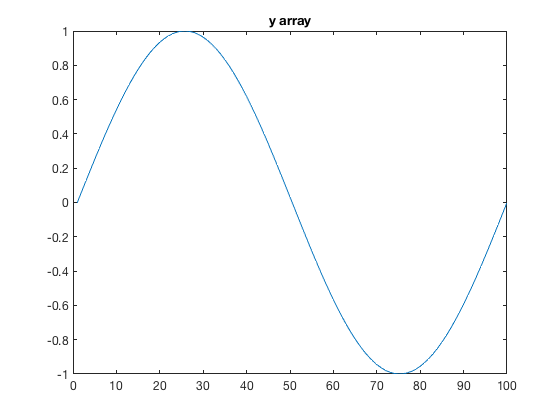# expand3 documentation

The expand3 function creates a 3D matrix from the product of a 2D grid and a 1D vector.

## Syntax

`Z3 = expand3(Z,y)`

## Description

Z3 = expand3(Z,y) creates a 3D matrix Z3 through elementwise multiplication of the 2D grid Z and the 1D array y.

## Examples

Imagine you have a 50x50 grid like this:

```Z = peaks(50);

pcolor(Z);
title 'Z grid'
```And you know that whole grid varies sinusoidally through time like this:

```% A sinusoid at 100 equally spaced intervals:
y = sin(linspace(0,2*pi,100));

plot(y)
title 'y array'
```These are the dimensions of Z and y:

```whos Z y
```
```  Name       Size             Bytes  Class     Attributes

Z         50x50             20000  double
y          1x100              800  double

```

We want to turn that 50x50 Z grid and the 1x100 y array into a 50x50x100 matrix where the first 50x50 slice of Z3 is equivalent to Z*y(1), the second slice is Z*y(2), etc. Here's how:

```Z3 = expand3(Z,y);

whos Z3
```
```  Name       Size                  Bytes  Class     Attributes

Z3        50x50x100            2000000  double

```

That's it.

You can then animate the plot and save it as a gif like this:

```% Plot the first frame:
h = surf(Z(:,:,1));
axis([-3 3 -3 3 -9 9])```
```% Make it fancy:
camlight
set(gca,'color','k')
set(gcf,'color','k')
caxis([min(Z(:)) max(Z(:))])```
```% Write the first frame:
gif('myfile.gif')```
```% Loop through each remaining frame:
for k = 2:100
set(h,'Zdata',Z(:,:,k))
gif % saves this frame
end```

And that's it. Here's what the final product looks like:## Author Info

This function is part of the Climate Data Toolbox for Matlab. The function and supporting documentation were written by Chad A. Greene of the University of Texas at Austin.